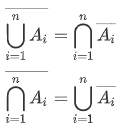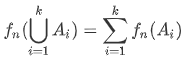## 1.样本点与样本空间

• 样本点

随机试验$E$的每个直接结果，常用$\omega$表示。

• 样本空间

$E$的全体样本点构成的集合，常用$\Omega$表示。

\begin{equation}
\Omega=\{\omega\}
\end{equation}

1. 样本空间是一个集合，它是由样本点这些元素构成的，可以用列举法或者描述法表示。

2. 在样本空间中，样本点数可以是有限个，也可以是无限个。

3. 在同样的试验条件下，试验目的不同，样本空间也不同。

## 2.随机事件

• 定义

试验E的样本空间$\Omega$的子集称为E的随机事件。随机时间简称事件，常用A、B、C等表示。每一个样本点都是一个基本事件。由多个样本点构成的事件为复合事件

• 必然事件

在每次试验中必然会发生的事件，用$\Omega$表示。样本空间是必然事件

• 不可能事件

在每次试验中都不可能发生的事件，用$\Phi$表示。空集是不可能事件

## 3.事件的关系

• 子事件($A\subset B$)

事件A发生一定导致B发生。

• 相等事件($A=B$)
\begin{equation}
(A=B)\iff\begin{cases}A\subset B \\ B\subset A\end{cases}
\end{equation}

• 和事件($A\cup B$)

A发生或者B发生。

• 积事件($A\cap B$$AB$)

A和B同时发生。

• 互斥(互不相容)事件($AB=\Phi$)

A和B不同时发生。当AB互斥时，可将$A\cup B$记为$A+B$

• 互逆(对立)事件

$(AB-\varnothing)\land (A\cup B=\Omega)$，A发生当且仅当B不发生。
A的对立事件记为$\overline A$

• 差事件(A-B)

$A-B=A-AB=A\overline{B}$，A发生而B不发生。

## 4.事件的运算

• 交换律
\begin{equation}
A\cup B=B\cup A, A\cap B=B\cap A
\end{equation}

• 结合律
\begin{equation}
A\cup(B\cup C)=(A\cup B)\cup C, A\cap(B\cap C)=(A\cap B)\cap C
\end{equation}

• 分配律
\begin{equation}
A\cup(B\cap C)=(A\cup B)\cap(A\cup C), A\cap(B\cup C)=(A\cap B)\cup(A\cap C)
\end{equation}

• 对偶律(德摩根定律)
\begin{equation}
\overline{A\cup B}=\overline{A}\cap\overline{B}, \overline{A\cap B}=\overline{A}\cup\overline{B}
\end{equation}## 5.概率的定义

• 频率的定义

在相同的条件下，进行了n次试验，如果事件A在者n次重复试验中出现了$n_A$次，则称比值$\frac{n_A}{n}$为事件A发生的频率。
\begin{equation}
f_n(A)=\frac{n_A}{n}
\end{equation}
频率反映了随机事件A发生的频繁程度。

• 概率的统计定义

事件A发生的频率稳定值p，成为A发生的概率，记为P(A)。

当n充分大时，可以用频率作为概率的近似值，这种确定概率的方法称为频率方法。

• 频率的性质

1. 非负有界性
\begin{equation}
0\le f_n(A)\le 1
\end{equation}

2. 规范性
\begin{equation}
f_n(\Omega)=1
\end{equation}

3. 有限可加性
$A_1,A_2,...A_k$是一组两两互斥的事件:• 概率的公理化定义

$\Omega$随机试验E的样本空间，对于该随机试验的每一个事件A赋予一个实数，记为$P(A)$，成为事件A的概率，如果集合函数$P(·)$满足下列公理:

1. 非负有界性
\begin{equation}
0\le P(A)\le 1
\end{equation}

2. 规范性
\begin{equation}
P(\Omega)=1
\end{equation}

3. 可列可加性
若A_1,A_2,…两两互斥:
\begin{equation}
P(A_1\cup A_2\cup …)=P(A_1)+P(A_2)+…
\end{equation}
公理3对于有限个两两互斥的事件仍然成立。

## 6.概率的性质

• 性质1:

\begin{equation}
P(\varnothing)=0
\end{equation}

• 性质2:
对任意A，有:
\begin{equation}
P(A)=1-P(\overline{A})
\end{equation}

• 性质3(减法公式):
对任意A,B有:
\begin{equation}
P(A-B)=P(A)-P(AB)
\end{equation}

• 性质4(广义加法公式):
\begin{equation}
P(A\cup B)=P(A)+P(B)-P(AB)\
\end{equation}

• 推论1:
\begin{equation}
A\supset B\to P(A-B)=P(A)-P(B)
\\
P(A\cup B\cup C)=P(A)+P(B)+P(C)-P(AB)-P(AC)-P(BC)+P(ABC)
\end{equation}

• 推论2:
\begin{equation}
A\supset B\to P(A)\ge P(B)
\end{equation}

## 7.古典概型

• 古典概型的特征
有限性: 试验的样本空间只含有限个样本点(基本事件)。
等可能性: 试验中每个基本事件等可能发生。

• 古典概率计算公式
设随机试验$E$为古典概型，其样本空间为$\Omega$对于任意事件$A$$m$$A$中所含样本总数，$n$$\Omega$中所含样本总数，有:
\begin{equation}
P(A)=\frac{m}{n}
\end{equation}

## 8.几何概型

• 特征
1.样本空间$\Omega$又无限个样本点。
2.每个样本点对应的基本事件的发生是等可能的。

• 定义
设随机试验$E$的每个结果是等可能地落入区域$\Omega$上地一点$M$，且$D\subset \Omega$，则点落入区域$D$上的概率为$P(A)=\frac{D的测度}{\Omega 的测度}$为事件$A$发生的概率，叫几何概率。

## 发送评论编辑评论

|´・ω・)ノ
ヾ(≧∇≦*)ゝ
(☆ω☆)
（╯‵□′）╯︵┴─┴
￣﹃￣
(/ω＼)
∠( ᐛ 」∠)＿
(๑•̀ㅁ•́ฅ)
→_→
୧(๑•̀⌄•́๑)૭
٩(ˊᗜˋ*)و
(ノ°ο°)ノ
(´இ皿இ｀)
⌇●﹏●⌇
(ฅ´ω`ฅ)
(╯°A°)╯︵○○○
φ(￣∇￣o)
ヾ(´･ ･｀｡)ノ"
( ง ᵒ̌皿ᵒ̌)ง⁼³₌₃
(ó﹏ò｡)
Σ(っ °Д °;)っ
( ,,´･ω･)ﾉ"(´っω･｀｡)
╮(╯▽╰)╭
o(*////▽////*)q
＞﹏＜
( ๑´•ω•) "(ㆆᴗㆆ)

Emoji